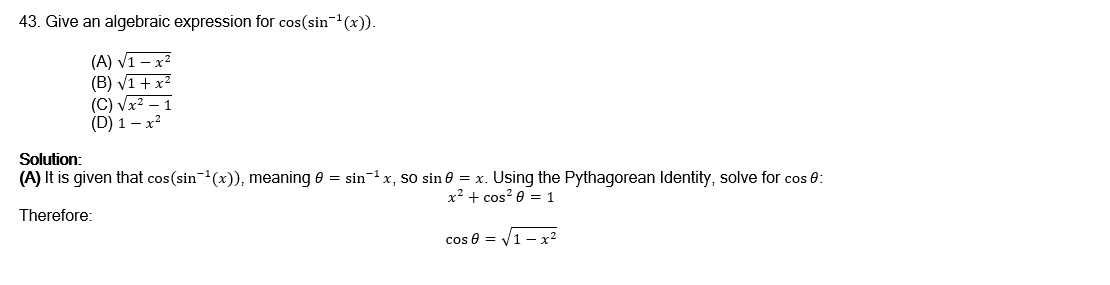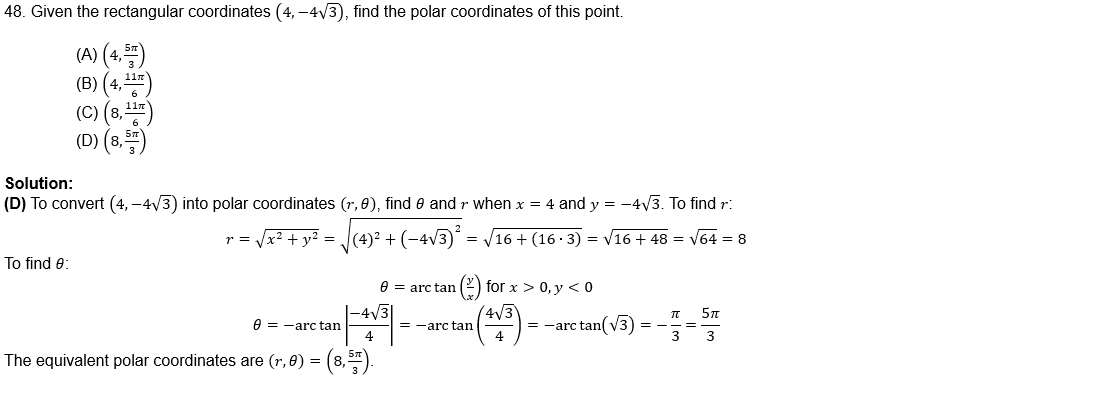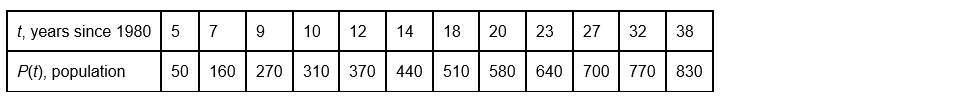Since the publication of Barron’s AP Precalculus Premium, 2024, the College Board has updated the number of multiple-choice questions in Section 1, Part A of the exam.

Here is what you need to know:NOTE: The online tests that accompany your book have been updated to reflect the current exam format.

For the latest information on the AP Precalculus course and exam visit the College Board Precalculus webpage.

The following questions can be eliminated from the practice tests in the book to reflect the 2024 AP Precalculus exam:

Practice Test 1, Section I

2, 7, 14, 26, 27, 29, 32, 33

Because the exam does not test on Unit 4 topics, Question 43 can be replaced with the following:Practice Test 2, Section I

14, 16, 19, 21, 27, 31, 32, 36

Because the exam does not test on Unit 4 topics, Question 48 can be replaced with the following:Section II

As noted above, because Unit 4 topics are not included in the exam, replace Section II, Question 1 with the following:

1. A study was performed in a local school district of a small town. The population of the student body from this district can be found in the table below.(a) Write a logarithmic regression model for the given data, rounding all coefficients to the nearest thousandth.

(b) Explain why a logarithmic regression model is appropriate to use in the context of the problem.

(c) Using the regression model from part (a), approximate the population in the year 1995 to the nearest tenth. Comment on the reasonableness of this prediction.

(d) Using the regression model from part (a), approximate the population in the year 2024 to the nearest tenth. Comment on the reasonableness of this prediction.

Solution

(a) Use technology to enter the data into L1 and L2, and calculate the logarithmic regression model using STAT CALC 9:LnReg. The regression model is y = −591.427 + 390.404 ln x

(b) The coefficient of determination is r2 ≈ 0.99816. Using a logarithmic regression model is appropriate since the population seems to increase rather rapidly in the beginning but then begins to level off although still increasing.

(c) To approximate the population in the year 1995, evaluate P(15) = −591.427 + 390.404 ln(15) ≈ 465.8. This seems reasonable as it fits within the data values between t = 14 and t = 18.

(d) To approximate the population in the year 2024, evaluate P(44) = −591.427 + 390.404 ln(44) ≈ 885.9. It is difficult to comment on the reasonableness of data outside of the provided domain. There are limiting factors on population and no guarantee that the population will continue to increase as it does with the provided data.

Note on Unit 4: Functions Involving Parameters, Vectors, and Matrices

While this unit is not included on the exam, we have chosen to include this chapter in the book to provide comprehensive support in Precalculus for students and for teachers who may include these topics in their course based on state or local requirements.